# Taylor series

A Taylor series, called after the English mathematician Brook Taylor (1685–1731), is an infinite sum of polynomial terms to approximate a function in the region about a certain point a. This is only possible if the function is behaving analytically in this neighbourhood. Such series about the point a = 0 are known as Maclaurin series, after the Scottish mathematician Colin Maclaurin (1698–1746). They work by ensuring that the approximate series matches up to the nth derivative of the function being approximated when it is approximated by a polynomial of degree n.

## Series

### General formula

An intuitive explanation of the Taylor series is that, in order to approximate the value of f(x), as a first approximation we use the value at another point a, i.e. f(a). If x and a are close together and f varies only slowly, this can be a good approximation. Then we refine the approximation step by step. The derivative of f is used to calculate approximately how much f would be expected to change between a and x, and this amount is added as a correction. But we assume we only know the derivative of f at a, and the derivative may change between the two numbers, so another correction is needed, involving the second derivative which is a measure of how much the first derivative changes. So it continues, adding corrections to corrections, and in the limit, if it converges then it converges to the actual value of f(x) even if x and a are far apart.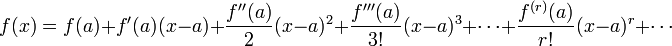$f(x)=f(a)+f'(a)(x-a)+\frac{f''(a)}{2}(x-a)^2+\frac{f'''(a)}{3!}(x-a)^3+\cdots+\frac{f^{(r)}(a)}{r!}(x-a)^r+\cdots$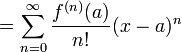$=\sum_{n=0}^{\infin} \frac{f^{(n)}(a)}{n!} (x-a)^{n}$

where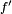$f'$ is the first derivative of the function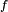$f$, and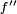$f''$ is the second derivative, and so on. Most practically used smooth functions satisfy this formula but not all of them. The smooth function f(x) = exp( − 1 / x2) (with f(0) = 0) has all derivatives at the origin equal to zero, but the function itself is not equal to zero. In order to prove Taylor formula for particular functions, mathematicians reduce the problem to proving Taylor formula for basic functions using a variety of theorems. Taylor formula for basic functions can be proved directly by estimating their derivatives.

## Convergence of the Taylor series and examples

The region of convergence of the Taylor series is always a circle. This circle is centered at the point of expansion. Radius of this circle is equal to the distance from this point to the closest singularity. Within the circle of convergence, the Taylor series can be used to make the polynomial approximation of holomorphic functions, just truncating the series.

###  Example: f(z)=1/z

A simple function, which is not polynomial, is f(z) = 1 / z This function is shown in the left hand side of the figure in the complex z plane with lines of constant real part and lines of constant image part. The polynomial approximation of this function can be obtained by truncating the Taylor series; for example, taking 21 terms of the Taylor series developed at z = 1 gives the approximation$t_{20}(z)=\sum_{n=0}^{20} (1-z)^n$

This function is shown in the right hand side of the figure. In the central part of the circle of convergence, the truncated Taylor series approximates the function.

Below, more examples of Taylor series of various functions are suggested.

### Exponential & Logarithmic functions$e^x=1+x+\frac{x^2}{2!}+\frac{x^3}{3!}+\cdots+\frac{x^r}{r!}+\cdots \qquad \forall x \in \mathbb{C}$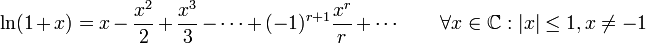$\ln(1+x)=x-\frac{x^2}{2}+\frac{x^3}{3}-\cdots+(-1)^{r+1}\frac{x^r}{r}+\cdots \qquad \forall x \in \mathbb{C}: |x|\le 1 , x\ne -1$

### Trigonometric functions$\sin x=x-\frac{x^3}{3!}+\frac{x^5}{5!}-\cdots+(-1)^{r}\frac{x^{2r+1}}{(2r+1)!}+\cdots \qquad \forall x$$\cos x=1-\frac{x^2}{2!}+\frac{x^4}{4!}+\cdots+(-1)^{r}\frac{x^{2r}}{(2r)!}+\cdots \qquad \forall x$$\tan x=x+\frac{x^3}{3}+\frac{2 x^5}{15}+\cdots+\frac{B_{2r} (-4)^r (1-4^r)}{(2r)!} x^{2r-1}+\cdots \qquad |x|<\frac{\pi}{2}$
where Bk=kth Bernoulli number.

### Inverse trigonometric functions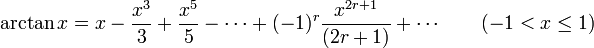$\arctan x=x-\frac{x^3}{3}+\frac{x^5}{5}-\cdots+(-1)^{r}\frac{x^{2r+1}}{(2r+1)}+\cdots \qquad (-1 < x \le 1)$

### Hyperbolic functions$\sinh x=x+\frac{x^3}{3!}+\frac{x^5}{5!}+\cdots+\frac{x^{2r+1}}{(2r+1)!}+\cdots \qquad \forall x$$\cosh x=1+\frac{x^2}{2!}+\frac{x^4}{4!}+\cdots+\frac{x^{2r}}{(2r)!}+\cdots \qquad \forall x$

### Inverse hyperbolic functions$\operatorname{Atanh} \, x=x+\frac{x^3}{3}+\frac{x^5}{5}+\cdots+\frac{x^{2r+1}}{(2r+1)}+\cdots \qquad (-1 < x \le 1)$Some content on this page may previously have appeared on Citizendium.